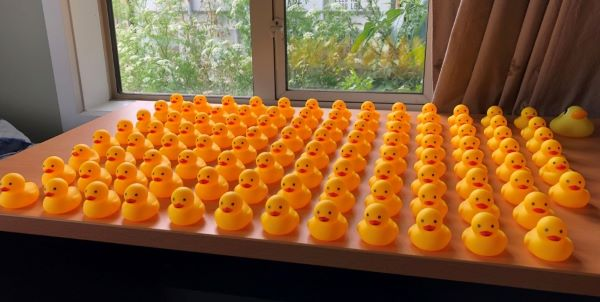Training Site# More Ducks in a Row

Input: Standard Input (stdin)
Output: Standard Output (stdout)
Memory limit: 126 megabytes
Time limit: 1.0 seconds

Chris loves ducks, but Thomas loves ducks even moreIn fact, Thomas has so many ducks that he has trouble getting them into a row.

Thomas's ducks are all kept on his desk, which is infinitely sized (that's how he can collect so many ducks). The desk is evenly divided into rows and columns. The rows are numbered like $0, 1, 2$ and so on, with row 0 being the bottom-most row. The columns are also numbered like $0, 1, 2$ and so on, with column 0 being the left-most column. Each row and column is exactly one unit wide.

Each of his ducks are located at a specific row and column. Additionally, all of his ducks are digital ducks (the same ones that Grant gave to Chris) so multiple ducks can occupy the same spot, and can move through or into each other without bumping.

Thomas wants to move all his ducks into a single row or column. Your task is to decide for Thomas which row or column he should move the ducks into. Once you have decided on a row or column, Thomas will move each duck the shortest distance possible to a spot on that row or column. It doesn't matter to Thomas whether you choose a row or you choose a column - either is fine. However, you should choose the row or column that minimizes the total distance Thomas has to move the ducks.

Thomas offers you a deal - if you can get all his ducks into the best possible row or column, he will reward you lavishly with 100 points towards your NZIC score. Are you willing to take up this quacking good deal?

## Input

• The first line will contain the number of ducks, $N$.
• $1 \le N \le 60,000$
• Each of the following $N$ lines represents the location of a duck. It will contain two integers, $c$ and $r$. Here, $c$ is the column the duck is in and $r$ is the row the duck is in.
• $0 \le c, r \le 1,000,000,000$ for every duck

## Output

Output a single integer - the minimum total distance required for Thomas to move the ducks into a single row or column.

• Subtask 1 (+30%): $0 \le c, r \le 400$ for every duck, and $N=2$.
• Subtask 2 (+40%): $0 \le c, r \le 400$ for every duck, and $1 \le N \le 200$
• Subtask 3 (+30%): No further constraints apply.

## Sample Explanation

### Sample 1

In this sample, the two ducks are at (column 0, row 1) and (column 4, row 4). We can choose row number 2. Thomas will then move the lower duck up one unit, and the top duck down two units. Therefore the total distance is $1+2=3$. Choosing any other row or column will not give a smaller distance, so this is our answer.

### Sample 2

In this sample, one optimal choice would be column number 3. Thomas will then move the leftmost duck 3 units right, not move the second-to-leftmost duck, and move the rightmost two ducks 1 and 3 units left. Thus the answer is 7. Note that two rightmost ducks will end up at the same position (column 3, row 6) but this is ok - these are digital ducks!

## Note:

If you are using a type sensitive language such as C, C++, C#, or Java then be sure to use variables, functions, and methods that support 64-bit precision. The answer does not exceed the maximum value of a signed 64-bit integer in any test case.

• ### Sample Input 1

2
4 4
0 1


### Sample Output 1

3

• ### Sample Input 2

4
0 1
3 3
4 6
6 6


### Sample Output 2

7